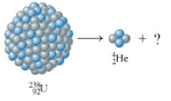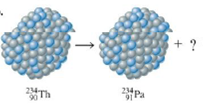Chapter 19, Problem 13E

Chapter
Section
Textbook Problem

# Supply the missing particle, and state the type of decay for each of the following nuclear processes.a.b.(a)

Interpretation Introduction

Interpretation: The missing particles and the type of decay for each of the given nuclear processes are to be stated.

Concept introduction: Nuclei of radioactive element decompose in various ways. There are two major categories. One involves a change in mass number of the decaying nucleus, while others do not. Types of radioactive processes include α particle production, β particle production, γ ray production, electron capture and many others.

To determine: The missing particle and the type of radioactive decay.

Explanation

The missing particle is 90234Th and the type of radioactive decay is alpha (α) decay.

The incomplete equation is as follows.

92238U24He+?

Since, the production of helium nucleus is characterized by alpha decay, hence the above process corresponds to alpha (α) decay

(b)

Interpretation Introduction

Interpretation: The missing particles and the type of decay for each of the given nuclear processes are to be stated.

Concept introduction: Nuclei of radioactive element decompose in various ways. There are two major categories. One involves a change in mass number of the decaying nucleus, while others do not. Types of radioactive processes include α particle production, β particle production, γ ray production, electron capture and many others.

To determine: The missing particle and the type of radioactive decay.

### Still sussing out bartleby?

Check out a sample textbook solution.

See a sample solution

#### The Solution to Your Study Problems

Bartleby provides explanations to thousands of textbook problems written by our experts, many with advanced degrees!

Get Started